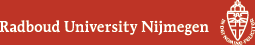# Wouter Cames van Batenburg### This website is (or will be) outdated. I moved to the Université Libre de Bruxelles as of April 1st 2018.

I am/was a PhD student in mathematics at Radboud University Nijmegen. My main research interests are in probability and combinatorics. In particular: colo(u)ring problems and extremal combinatorics on graphs, as well as percolation theory.

## In preparation

• W. Cames van Batenburg, R.J. Kang and F. Pirot. Strong cliques and forbidden cycles.
• W. Cames van Batenburg, T. Huynh, G. Joret and J.-F. Raymond. A tight Erdős-Pósa function for planar minors.

## Some questions that interest/haunt me.

• BEC-conjecture (1976). Let G_1 and G_2 be two graphs on n vertices with maximum degrees \Delta_1 and \Delta_2, respectively. Show that G_1 and G_2 pack if (\Delta_1+1)(\Delta_2+1) <= n+1. See my paper with Ross Kang for more information.
• Nontrivial percolation threshold. Given a graph G with isoperimetric dimension strictly larger than 1, does it hold that the percolation threshold of G is strictly less than 1? This question traces back to the paper Percolation Beyond Z^d, Many Questions And a Few Answers and there has been quite some progress for transitive and/or planar graphs. A more modest and related question that may be tractable: given a graph G with bounded degree and isoperimetric dimension larger than 2, does there exist a uniform constant C>0 such that for each nonempty subset A of V(G), the size of the edge boundary of A (with respect to G) is at least C times the diameter of the graph induced by A?
• Variance in Last Passage Percolation. Put iid random weights on each vertex of Z^2. Consider oriented (North-East) paths of length n that start in (0,0). The weight of a path is the sum of the weights of the vertices that are visited by the path. The heaviest path is the path with the largest weight, among all paths of length n. Does it hold that the variance of the weight of the heaviest path is asymptotically equal to a constant multiple of n^{2/3}, as n goes to infinity? This (and more) is known to be true if the distribution of the vertex weights is either an exponential or a geometric distribution, but it is expected to hold for a much wider class of distributions. In general, roughly, the variance is only known to be between log(n) and n/log(n). See this survey for more details.
• Hard squares constant. (Warning: dangerous problem). Let N and n be positive integers. Consider the set A(n,N) of all 0,1-words of length n for which there are no pairs of 1's that are adjacent or at distance exactly N. Find a nice exact expression for \lim_{N \rightarrow \infty} \lim_{n \rightarrow \infty} |A(n,N)|^{1/n}. This limit exists and it is equal to the so-called Hard squares constant, of which many digits are semi-rigorously known.

## Teaching

As a student at Leiden University (until 2013) and then as a PhD student at Radboud University, I have taught exercise classes for the following courses:

• Autumn 2017 and Autumn 2016, Advanced Probability.
• Spring 2017, Game theory.
• Spring 2016 and Spring 2015, Inleiding Kansrekening (Introduction to probability theory).
• Autumn 2015, Autumn 2014 and Spring 2014, Applied Stochastics (Random graphs and Ramsey theory).
• Autumn 2012 and Autumn 2010, Analyse 1 (Calculus).
• Spring 2011, Analyse 4 (Complex analysis).
• Spring 2010, Analyse 2 (Advanced calculus).
• Autumn 2010, Calculus A (Basic calculus for the Biopharmaceutical Sciences).

## Other

• W. Cames van Batenburg, A. Czechowski, J. van den Leer Duran, B. Lindenhovius and E. Siero. Frequency decompositions in autoregression models. For the Dutch Study Group Mathematics with Industry 2016, 13pp.

Last update: May 27, 2018